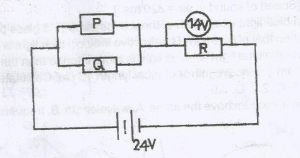A battery of emf 24V is connected to three resistor P, Q, and R as illustrated in the given diagram. Determine the voltage across the resistor Q.

A. 5V
B. 10V
C. 14V
D. 24V

Correct Answer: Option B

B. 10V

Explanation

E = V1 + V2

24V = 14V + VQP

VQP = 24 – 14V

VQP = 10V